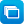# Mathematics / Year 6 / Number and Algebra / Number and place value

Curriculum content descriptions

Identify and describe properties of prime, composite, square and triangular numbers (ACMNA122)

Elaborations
• understanding that some numbers have special properties and that these properties can be used to solve problems
• representing composite numbers as a product of their prime factors and using this form to simplify calculations by cancelling common primes
• understanding that if a number is divisible by a composite number then it is also divisible by the prime factors of that number (for example 216 is divisible by 8 because the number represented by the last three digits is divisible by 8, and hence 216 is also divisible by 2 and 4)
General capabilities
• Literacy Literacy
• Numeracy Numeracy
ScOT terms

Triangular numbers,  Composite numbers,  Perfect squares,  Prime numbers

## Refine results by

Year level 5-6
Resource type
Learning area Mathematics

## Refine by topic

Related topic### Sites2See – number for primary

Selected links to a range of interactive online resources for the study of number in Foundation to Year 6 Mathematics.### Circus towers: square stacks

Work out how many acrobats are needed to form square-shaped human towers. Start by building a square tower with four acrobats: two acrobats in the base layer and two acrobats standing on their shoulders. Examine a table and graph of the total number of acrobats in the towers. Predict the number of acrobats needed to build ...### What are factors?

What are factors? Watch as the jelly babies in this clip show you! What are the factors of 12? How many factors does the number 11 have? Try explaining to a friend what a prime number is.### Prime, composite and square numbers

These resources and tasks focus on prime and composite numbers and can be used to support an inquiry into prime numbers and factors.### Patterns, primes and Pascal's Triangle

Are you intrigued by patterns? Check out Vi Hart as she explains how to visualise patterns in prime numbers, using Ulam's Spiral. Watch as Vi creates patterns, using Pascal's Triangle to explore relationships in number. See what happens when she circles the odd numbers. What rule does she use to create the final pattern?### Fascinating prime numbers

Use this teaching resource to investigate prime numbers with your students. Go back in time to the ancient Greeks (around 300 BC) to find out how Euclid demonstrated that there is an infinite number of primes. Investigate ways to identify prime numbers using algebra and square numbers and how to represent large primes using ...### TIMES Module 19: Number and Algebra: multiples, factors and powers - teacher guide

This is a 33-page guide for teachers. It introduces the concepts of primes, composites, prime factorisation, factors, multiples, odd and even numbers, the least common multiple and the highest common factor.### The beauty of prime numbers

A prime number is a number that only has two factors: one and itself. Listen to Adam Spencer and Richard Glover discussing prime numbers. They cover how we define these numbers and how and why prime numbers are widely used in internet encryption.### Palindromic numbers

In a grade 6 mathematics class a teacher plans for, delivers and reflects on a lesson focusing on Number and Algebra. In planning the lesson, she articulates a preference for using open-ended problem-solving activities and resources that can cater for a range of abilities. In designing activities, and in selecting resources, ...### Prime numbers and unbreakable codes

Imagine if anyone was able to read all our secret, encrypted messages and information. Watch and find out how scientists at the Australian National University are developing a new encryption system using quantum physics and quantum computing.### Prime number keys

Have you ever wondered how modern day encryption works? How are messages and financial transactions kept hidden from cyber criminals and hackers? Listen to reporter Ruben Meerman and mathematician Simon Pampena discuss the largest prime number ever found and how prime numbers are used to encrypt electronic information.### Mental calculation strategies - addition and subtraction

This collection of 21 digital curriculum resources focuses on assisting students to develop efficient and effective mental calculation strategies for performing additions and subtractions of two-, three- and four-digit whole numbers. It is organised in three categories: developing strategies - addition; developing strategies ...